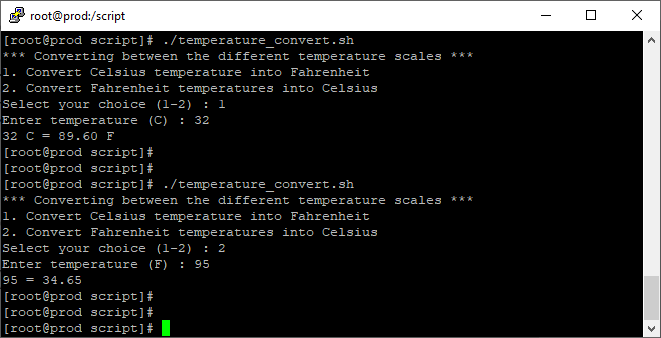Search
•Arun Kumar

# Shell Script to Convert Temperature

Linux shell script allows the user to enter the temperature and provides the option to convert in Fahrenheit or Celsius. To achieve this we will use for loop. A ‘for loop’ is a bash programming language statement that allows code to be repeatedly executed.

`vi temperature_convert.sh`
```#!/bin/bash

echo "*** Converting between the different temperature scales ***"
echo "1. Convert Celsius temperature into Fahrenheit"
echo "2. Convert Fahrenheit temperatures into Celsius"
echo -n "Select your choice (1-2) : "

if [ \$choice -eq 1 ]
then
echo -n "Enter temperature (C) : "
# formula Tf=(9/5)*Tc+32
tf=\$(echo "scale=2;((9/5) * \$tc) + 32" |bc)
echo "\$tc C = \$tf F"
elif [ \$choice -eq 2 ]
then
echo -n "Enter temperature (F) : "
# formula Tc=(5/9)*(Tf-32)
tc=\$(echo "scale=2;(5/9)*(\$tf-32)"|bc)
echo "\$tf = \$tc"
else
echo "Please select 1 or 2 only"
exit 1
fi ```

Here is the sample output of the above script## More ways to use for loop

• You can use 'for' like the C programming language.

• You can use the Range of numbers after the “in” keyword.

753 views

See All